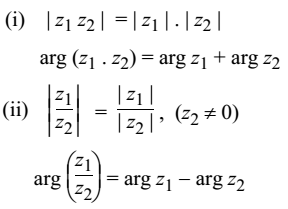# Polar Form of Complex Number

Let (r, θ) be the polar co-ordinates of the point.

P = P(x, y) in the complex plane corresponding to the complex number z = x + iy.x = r cos θ

y = r sin θ

r = √(x2 + y2) = |x + iy| is called the modulus or the absolute value of z denoted by |z|.

tan θ = y/x

θ is called the amplitude or argument of z = x + iy denoted by amp z or arg z and is measured as the angle which the line OP makes with the positive x-axis (in the anti clockwise sense).

z = x + iy = r(cosθ + i sin θ) is called the polar form or the modulus amplitude form of the complex number.

You can also use the abbreviation cis θ for cos θ + i sin θ.

### Principal Value

The argument of z is not unique. Any two distinct arguments of z differ from each other by an integral multiple of 2π. In order to specify a unique value of arg z, you may restrict its value to some interval of length 2π.

For an arbitrary z ≠ 0 the principal value of arg z is defined to be the unique value of z that satisfies −π < arg z ≤ π.

### Properties